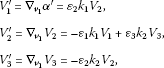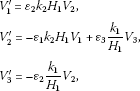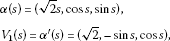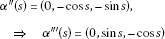# The tangent spherical image and ccr-curve of a time-like curve in ${\mathbb{L}}^{3}$

## Abstract

In this work, we define the tangent spherical image of a unit speed time-like curve lying on the pseudohyperbolic space ${H}_{0}^{2}\left(r\right)$ in ${\mathbb{L}}^{3}$. In addition, we calculate a ccr-curve of this curve in ${\mathbb{L}}^{3}$. Besides, we determine a relation between harmonic curvature and a ccr-curve in ${\mathbb{L}}^{3}$, and so we obtain some new results.

## Introduction

Let $X=\left({x}_{1},{x}_{2},{x}_{3}\right)$ and $Y=\left({y}_{1},{y}_{2},{y}_{3}\right)$ be two non-zero vectors in the three-dimensional Lorentz-Minkowski space ${\mathbb{R}}_{1}^{3}$. We denoted ${\mathbb{R}}_{1}^{3}$ shortly by ${\mathbb{L}}^{3}$. For $X,Y\in {\mathbb{L}}^{3}$,

$〈X,Y〉=-{x}_{1}{y}_{1}+{x}_{2}{y}_{2}+{x}_{3}{y}_{3}$

is called a Lorentzian inner product. The couple $\left\{{\mathbb{R}}_{1}^{3},〈\phantom{\rule{0.25em}{0ex}},\phantom{\rule{0.25em}{0ex}}〉\right\}$ is called a Lorentzian space and denoted by ${\mathbb{L}}^{3}$. Then the vector X of ${\mathbb{L}}^{3}$ is called

1. (i)

time-like if $〈X,X〉<0$,

2. (ii)

space-like if $〈X,X〉>0$ or $X=0$,

3. (iii)

a null (or light-like) vector if $〈X,X〉=0$, $\phantom{\rule{0.25em}{0ex}}X\ne 0$.

The norm of a vector X is given by $\parallel X\parallel =\sqrt{|〈X,X〉|}$. Therefore, X is a unit vector if $〈X,X〉=±1$. Next, vectors X, Y in ${\mathbb{L}}^{3}$ are said to be orthogonal if $〈X,Y〉=0$. The velocity of a curve $\alpha \left(s\right)$ is given by $\parallel {\alpha }^{\mathrm{\prime }}\left(s\right)\parallel$. Space-like or time-like $\alpha \left(s\right)$ is said to be parametrized by an arclength function s if $〈{\alpha }^{\mathrm{\prime }}\left(s\right),{\alpha }^{\mathrm{\prime }}\left(s\right)〉=±1$ . For any $X=\left({x}_{1},{x}_{2},{x}_{3}\right)$, $Y=\left({y}_{1},{y}_{2},{y}_{3}\right)\in {\mathbb{R}}_{1}^{3}$, the pseudo-vector product of a X and Y is defined as follows:

$X\mathrm{\Lambda }Y=\left(-\left({x}_{2}{y}_{3}-{x}_{3}{y}_{2}\right),{x}_{3}{y}_{1}-{x}_{1}{y}_{3},{x}_{1}{y}_{2}-{x}_{2}{y}_{1}\right)$

.

## 1 Basic concepts

Definition 1.1 An arbitrary curve $\alpha :I⟶{\mathbb{L}}^{3}$ in the space ${\mathbb{L}}^{3}$ can locally be space-like, time-like or a null curve if, respectively, all of its velocity vectors ${\alpha }^{\mathrm{\prime }}\left(s\right)$ are space-like, time-like or null .

Definition 1.2 Let $\alpha \subset {\mathbb{L}}^{3}$ be a given time-like curve. If the Frenet vector $\left\{{V}_{1}\left(s\right),{V}_{2}\left(s\right),{V}_{3}\left(s\right)\right\}$ which corresponds to $s\in I$ is defined as

${k}_{i}:I⟶\mathbb{R},\phantom{\rule{1em}{0ex}}{k}_{i}\left(s\right)=〈{V}_{i}^{\mathrm{\prime }}\left(s\right),{V}_{i+1}\left(s\right)〉,$

then the function ${k}_{i}$ is called an i th curvature function of the time-like curve α, and the real ${k}_{i}\left(s\right)$ is also called an i th curvature at the point $\alpha \left(s\right)$ .

Definition 1.3 Let $\alpha :I⟶{\mathbb{L}}^{3}$ be a unit speed non-null curve in ${\mathbb{L}}^{3}$. The curve α is called a Frenet curve of osculating order d ($d\le 3$) if its 3rd order derivatives ${\alpha }^{\mathrm{\prime }}\left(s\right)$, ${\alpha }^{\mathrm{\prime }\mathrm{\prime }}\left(s\right)$, ${\alpha }^{\mathrm{\prime }\mathrm{\prime }\mathrm{\prime }}\left(s\right)$ are linearly independent and ${\alpha }^{\mathrm{\prime }}\left(s\right)$, ${\alpha }^{\mathrm{\prime }\mathrm{\prime }}\left(s\right)$, ${\alpha }^{\mathrm{\prime }\mathrm{\prime }\mathrm{\prime }}\left(s\right)$, ${\alpha }^{ıv}\left(s\right)$ are no longer linearly independent for all $s\in I$. For each Frenet curve of order 3, one can associate an orthonormal 3-frame $\left\{{V}_{1}\left(s\right),{V}_{2}\left(s\right),{V}_{3}\left(s\right)\right\}$ along α (such that ${\alpha }^{\mathrm{\prime }}\left(s\right)={V}_{1}$) called the Frenet frame and ${k}_{1},{k}_{2}:I⟶\mathbb{R}$ called the Frenet curvatures, such that the Frenet formulas are defined in the usual way:where is the Levi-Civita connection of ${\mathbb{L}}^{3}$.

Definition 1.4 A non-null curve $\alpha :I⟶{\mathbb{L}}^{3}$ is called a W-curve (or helix) of rank3, if α is a Frenet curve of osculating order 3 and the Frenet curvatures ${k}_{i}$, $1\le i\le 2$, are non-zero constants.

## 2 Harmonic curvatures and constant curvature ratios in ${\mathbb{L}}^{3}$

Definition 2.1 Let α be a non-null curve of osculating order 3. The harmonic functions

${H}_{j}:I⟶\mathbb{R},\phantom{\rule{1em}{0ex}}0\le j\le 1,$

defined by

$\left\{\begin{array}{c}{H}_{0}=0,\hfill \\ {H}_{1}=\frac{{k}_{1}}{{k}_{2}}\hfill \end{array}$

are called harmonic curvatures of α, where ${k}_{1}$, ${k}_{2}$ are Frenet curvatures of α which are not necessarily constant.

Definition 2.2 Let α be a time-like curve in ${\mathbb{L}}^{3}$ with ${\alpha }^{\mathrm{\prime }}\left(s\right)={V}_{1}$. $Xϵ\chi \left({\mathbb{L}}^{3}\right)$ being a constant unit vector field, if

$〈{V}_{1},X〉=cosh\phi \phantom{\rule{1em}{0ex}}\left(\text{constant}\right),$

then α is called a general helix (inclined curves) in ${\mathbb{L}}^{3}$, φ is called a slope angle and the space $Sp\left\{X\right\}$ is called a slope axis .

Definition 2.3 Let α be a non-null of osculating order 3. Then α is called a general helix of rank1 if

${H}_{1}^{2}=c,$

holds, where $c\ne 0$ is a real constant.

We have the following results.

Corollary 2.1

1. (i)

If ${H}_{1}=0$, then α is a straight line.

2. (ii)

If ${H}_{1}$is constant, then α is a general helix of rank1.

Proof By the use of the above definition, we obtain the proof. □

Proposition 2.1 Let α be a curve in ${\mathbb{L}}^{3}$ of osculating order 3. Thenwhere ${H}_{1}$ is harmonic curvature of α.

Proof By using the Frenet formulas and the definitions of harmonic curvatures, we get the result. □

Now, we will give the relation between harmonic curvature and a ccr-curve in ${\mathbb{L}}^{3}$.

Definition 2.4 A curve $\alpha :I⟶{\mathbb{L}}^{3}$ is said to have constant curvature ratios (that is to say, it is a ccr-curve) if all the quotients ${\epsilon }_{i}\left(\frac{{k}_{i+1}}{{k}_{i}}\right)$ are constant, where ${\epsilon }_{i}=〈{V}_{i},{V}_{i}〉=±1$.

Corollary 2.2 For $i=1$, the ccr-curve is $\frac{{\epsilon }_{1}}{{H}_{1}}$.

Proof The proof can be easily seen by using the definitions of harmonic curvature and ccr-curve. □

Corollary 2.3 Let $\alpha :I⟶{\mathbb{L}}^{3}$ be a ccr-curve. If $\frac{{\epsilon }_{1}}{{H}_{1}}=c$, c is a constant, then ${\left(\frac{{\epsilon }_{1}}{{H}_{1}}\right)}^{\mathrm{\prime }}=0$.

Proof The proof is obvious. □

## 3 Tangent spherical image

Definition 3.1 

Let $n⩾2$ and $0\le v\le n$. Then the pseudohyperbolic space of radius $r>0$ in ${\mathbb{R}}_{1}^{3}$ is the hyperquadric

${H}_{0}^{2}\left(r\right)=\left\{p\in {\mathbb{R}}_{1}^{3}:〈p,p〉=-{r}^{2}\right\}$

with dimension 2 and index 0.

Definition 3.2 Let $\alpha =\alpha \left(s\right)$ be a unit speed time-like curve in ${\mathbb{L}}^{3}$. If we translate the tangent vector to the center 0 of the pseudohyperbolic space ${H}_{0}^{2}\left(r\right)$, we obtain a curve $\delta =\delta \left({s}_{\delta }\right)$. This curve is called the tangent spherical image of a curve α in ${\mathbb{L}}^{3}$.

Theorem 3.1 

1. (i)

Let $\alpha =\alpha \left(s\right)$ be a unit speed time-like curve and $\delta =\delta \left({s}_{\delta }\right)$ be its tangent spherical image. Then $\delta =\delta \left({s}_{\delta }\right)$ is a space-like curve.

2. (ii)

Let $\alpha =\alpha \left(s\right)$ be a unit speed time-like curve and $\delta =\delta \left({s}_{\delta }\right)$ be its tangent spherical image. If α is a ccr-curve or a helix (i.e. W-curve), then δ is also a helix.

Proof From  it is easy to see the proof of the theorem. □

## 4 An example

Example 4.1 Let us consider the following curve in the space ${\mathbb{L}}^{3}$:where $〈{\alpha }^{\mathrm{\prime }}\left(s\right),{\alpha }^{\mathrm{\prime }}\left(s\right)〉=-1$, which shows $\alpha \left(s\right)$ is a unit speed time-like curve. Thus $\parallel {\alpha }^{\mathrm{\prime }}\left(s\right)\parallel =1$. We express the following differentiations:and

${V}_{2}\left(s\right)=\frac{{\alpha }^{\mathrm{\prime }\mathrm{\prime }}\left(s\right)}{\parallel {\alpha }^{\mathrm{\prime }\mathrm{\prime }}\left(s\right)\parallel }={\alpha }^{\mathrm{\prime }\mathrm{\prime }}\left(s\right).$

So, we have the first curvature as

${k}_{1}\left(s\right)=〈{V}_{1}^{\mathrm{\prime }}\left(s\right),{V}_{2}\left(s\right)〉=1=\text{constant}.$

Moreover, we can write the third Frenet vector of the curve as follows:

${V}_{3}\left(s\right)={V}_{1}\left(s\right)\mathrm{\Lambda }{V}_{2}\left(s\right)=\left(-1,\sqrt{2}sins,-\sqrt{2}coss\right).$

Finally, we have the second curvature of $\alpha \left(s\right)$ as

${k}_{2}\left(s\right)=〈{V}_{2}^{\mathrm{\prime }}\left(s\right),{V}_{3}\left(s\right)〉=\sqrt{2}=\text{constant}.$

Now, we will calculate a ccr-curve of $\alpha \left(s\right)$ in ${\mathbb{L}}^{3}$. If the vector ${V}_{1}$ is time-like, then ${\epsilon }_{1}=-1$,

${\epsilon }_{1}\frac{{k}_{2}}{{k}_{1}}=-\sqrt{2}=\text{constant}.$

Thus $\alpha \left(s\right)$ is a ccr-curve in ${\mathbb{L}}^{3}$.

## References

1. O’Neill B: Semi-Riemannian Geometry with Applications to Relativity. Academic Press, New York; 1983.

2. Öğrenmiş AO, Balgetir H, Ergüt M:On the ruled surfaces in Minkowski 3-space ${\mathbb{R}}_{1}^{3}$. J. Zhejiang Univ. Sci. A 2006, 7(3):326–329. 10.1631/jzus.2006.A0326

3. Petrovic-Torgasev M, Sucurovic E: W-curves in Minkowski space-time. Novi Sad J. Math. 2002, 32(2):55–65.

4. Bektaş M, Ergüt M, Soylu D: The characterization of the spherical timelike curves in 3-dimensional Lorentzian space. Bull. Malays. Math. Soc. 1998, 21: 117–125.

5. Ekmekçi N, Hacisalihoglu HH, İlarslan K: Harmonic curvatures in Lorentzian space. Bull. Malays. Math. Soc. 2000, 23: 173–179.

6. Yılmaz S, Özyılmaz E, Yaylı Y, Turgut M:Tangent and trinormal spherical images of a time-like curve on the pseudohyperbolic space ${H}_{0}^{3}$. Proc. Est. Acad. Sci. 2010, 59(3):216–224. 10.3176/proc.2010.3.04

## Acknowledgements

Dedicated to Prof. Hari M. Srivastava.

## Author information

Authors

### Corresponding author

Correspondence to Esen İyigün.

### Competing interests

The author declares that they have no competing interests.

## Rights and permissions

Reprints and Permissions

İyigün, E. The tangent spherical image and ccr-curve of a time-like curve in ${\mathbb{L}}^{3}$. J Inequal Appl 2013, 55 (2013). https://doi.org/10.1186/1029-242X-2013-55

• Accepted:

• Published:

• DOI: https://doi.org/10.1186/1029-242X-2013-55

### Keywords

• Lorentzian Space
• Null Curve
• Arbitrary Curve
• Unit Vector Field
• Harmonic Curvature